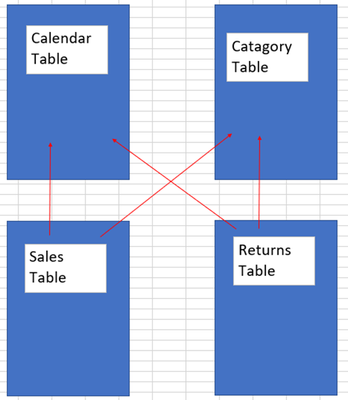cancel
Showing results for
Did you mean:Helper I

## Adding Custom Row to Matrix Table

Hi,

I'm looking to add some custom rows to a matrix table.

Currently I have been able to produce my data in the following format;However I would like to add a custom calculated row which is ratio of the category subtotals.However I would like to add a custom calculated row which is ratio of the category subtotals.

Data Model

I have a sales table and a returns table which are both connected to a lookup and calendar table.Any help would be appreciated.

1 ACCEPTED SOLUTIONCommunity Support

Hi @Peppearson ,

The data in row field in matrix should be loaded in data model, so "Components/Bikes"/"Accessories/Clothing" should be in a column. Here I suggest you to add a Flag column like [Compare Part] column in your table.

``````Compare Part =
IF('Table'[Category] in {"Bikes","Components"},"Components/Bikes","Accessories/Clothing")``````Measure:

``````M_Sales =
IF (
ISINSCOPE ( 'Table'[Subcategory] ),
CALCULATE ( SUM ( 'Table'[Sales] ) ),
IF (
ISINSCOPE ( 'Table'[Category] ),
CALCULATE ( SUM ( 'Table'[Sales] ) ),
IF (
ISINSCOPE ( 'Table'[Compare Part] ),
SWITCH (
SELECTEDVALUE ( 'Table'[Compare Part] ),
"Components/Bikes",
DIVIDE (
CALCULATE ( SUM ( 'Table'[Sales] ), 'Table'[Category] = "Components" ),
CALCULATE ( SUM ( 'Table'[Sales] ), 'Table'[Category] = "Bikes" )
),
"Accessories/Clothing",
DIVIDE (
CALCULATE ( SUM ( 'Table'[Sales] ), 'Table'[Category] = "Accessories" ),
CALCULATE ( SUM ( 'Table'[Sales] ), 'Table'[Category] = "Clothing" )
)
)
)
)
)``````

Result is as below.Best Regards,
Rico Zhou

If this post helps, then please consider Accept it as the solution to help the other members find it more quickly.Community Support

Hi @Peppearson ,

The data in row field in matrix should be loaded in data model, so "Components/Bikes"/"Accessories/Clothing" should be in a column. Here I suggest you to add a Flag column like [Compare Part] column in your table.

``````Compare Part =
IF('Table'[Category] in {"Bikes","Components"},"Components/Bikes","Accessories/Clothing")``````Measure:

``````M_Sales =
IF (
ISINSCOPE ( 'Table'[Subcategory] ),
CALCULATE ( SUM ( 'Table'[Sales] ) ),
IF (
ISINSCOPE ( 'Table'[Category] ),
CALCULATE ( SUM ( 'Table'[Sales] ) ),
IF (
ISINSCOPE ( 'Table'[Compare Part] ),
SWITCH (
SELECTEDVALUE ( 'Table'[Compare Part] ),
"Components/Bikes",
DIVIDE (
CALCULATE ( SUM ( 'Table'[Sales] ), 'Table'[Category] = "Components" ),
CALCULATE ( SUM ( 'Table'[Sales] ), 'Table'[Category] = "Bikes" )
),
"Accessories/Clothing",
DIVIDE (
CALCULATE ( SUM ( 'Table'[Sales] ), 'Table'[Category] = "Accessories" ),
CALCULATE ( SUM ( 'Table'[Sales] ), 'Table'[Category] = "Clothing" )
)
)
)
)
)``````

Result is as below.Best Regards,
Rico Zhou

If this post helps, then please consider Accept it as the solution to help the other members find it more quickly.